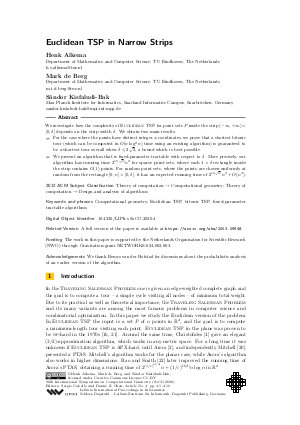Document# Euclidean TSP in Narrow Strips

### Authors Henk Alkema, Mark de Berg, Sándor Kisfaludi-Bak## File

LIPIcs.SoCG.2020.4.pdf
• Filesize: 0.89 MB
• 16 pages

## Acknowledgements

We thank Remco van der Hofstad for discussions about the probabilistic analysis of an earlier version of the algorithm.

## Cite As

Henk Alkema, Mark de Berg, and Sándor Kisfaludi-Bak. Euclidean TSP in Narrow Strips. In 36th International Symposium on Computational Geometry (SoCG 2020). Leibniz International Proceedings in Informatics (LIPIcs), Volume 164, pp. 4:1-4:16, Schloss Dagstuhl - Leibniz-Zentrum für Informatik (2020)
https://doi.org/10.4230/LIPIcs.SoCG.2020.4

## Abstract

We investigate how the complexity of {Euclidean TSP} for point sets P inside the strip (-∞,+∞)×[0,δ] depends on the strip width δ. We obtain two main results. - For the case where the points have distinct integer x-coordinates, we prove that a shortest bitonic tour (which can be computed in O(n log²n) time using an existing algorithm) is guaranteed to be a shortest tour overall when δ ⩽ 2√2, a bound which is best possible. - We present an algorithm that is fixed-parameter tractable with respect to δ. More precisely, our algorithm has running time 2^{O(√δ)} n² for sparse point sets, where each 1×δ rectangle inside the strip contains O(1) points. For random point sets, where the points are chosen uniformly at random from the rectangle [0,n]× [0,δ], it has an expected running time of 2^{O(√δ)} n² + O(n³).

## Subject Classification

##### ACM Subject Classification
• Theory of computation → Computational geometry
• Theory of computation → Design and analysis of algorithms
##### Keywords
• Computational geometry
• Euclidean TSP
• bitonic TSP
• fixed-parameter tractable algorithms

## Metrics

• Access Statistics
• Total Accesses (updated on a weekly basis)
0

## References

1. H Alkema, M. de Berg, and S. Kisfaludi-Bak. Euclidean TSP in narrow strips. arXiv, 2020. URL: http://arxiv.org/abs/2003.09948.
2. S. Arora. Polynomial time approximation schemes for Euclidean traveling salesman and other geometric problems. J. ACM, 45(5):753-782, 1998. URL: https://doi.org/10.1145/290179.290180.
3. H. L. Bodlaender, M. Cygan, S. Kratsch, and J. Nederlof. Deterministic single exponential time algorithms for connectivity problems parameterized by treewidth. Inf. Comput., 243:86-111, 2015. URL: https://doi.org/10.1016/j.ic.2014.12.008.
4. N. Christofides. Worst-case analysis of a new heuristic for the travelling salesman problem. Technical report, Graduate School of Industrial Administration, Carnegie Mellon University, 1976.5. T.H. Cormen, C.E. Leiserson, R.L. Rivest, and C. Stein. Introduction to Algorithms (3rd edition). MIT Press, 2009.6. M. Cutler. Efficient special case algorithms for the n-line planar traveling salesman problem. Networks, 10:183-195, 1980. URL: https://doi.org/10.1002/net.3230100302.
7. M. Cygan, S. Kratsch, and J. Nederlof. Fast hamiltonicity checking via bases of perfect matchings. J. ACM, 65(3):12:1-12:46, 2018. URL: https://doi.org/10.1145/3148227.
8. M. de Berg, H.L. Bodlaender, S. Kisfaludi-Bak, and S. Kolay. An ETH-tight exact algorithm for Euclidean TSP. In Proc. 59th IEEE Symp. Found. Comput. Sci. (FOCS), pages 450-461, 2018. URL: https://doi.org/10.1109/FOCS.2018.00050.
9. M. de Berg, K. Buchin, B.M.P. Jansen, and G. Woeginger. Fine-grained complexity analysis of two classic TSP variants. In Proc. 43rd Int. Conf. Automata Lang. Prog. (ICALP), pages 5:1-5:14, 2016. URL: https://doi.org/10.4230/LIPIcs.ICALP.2016.5.
10. M. de Berg, O. Cheong, M. van Kreveld, and M. Overmars. Computational Geometry: Algorithms and Applications. Springer, 2008. URL: https://doi.org/10.1007/978-3-540-77974-2.
11. V.G. Deineko, M. Hoffmann, Y. Okamoto, and G.J. Woeginger. The traveling salesman problem with few inner points. Oper. Res. Lett., 34(1):106-110, 2006. URL: https://doi.org/10.1016/j.orl.2005.01.002.
12. V.G. Deineko, R. van Dal, and G. Rote. The convex-hull-and-line traveling salesman problem: a solvable case. Inf. Proc. Lett., 51:141-148, 1994. URL: https://doi.org/10.1016/0020-0190(94)00071-9.
13. V.G. Deineko and G. Woeginger. The convex-hull-and-k-line traveling salesman problem. Inf. Proc. Lett., 59(3):295-301, 1996. URL: https://doi.org/10.1016/0020-0190(96)00125-1.
14. J. Doe. Probability inequalities for sums of bounded random variables. The Collected Works of Wassily Hoeffding, pages 409-426, 1994. URL: https://doi.org/10.1007/978-1-4612-0865-5_26.
15. H. Edelsbrunner, G. Rote, and E. Welzl. Testing the necklace condition for shortest tours and optimal factors in the plane. Theoret. Comput. Sci., 66:157-180, 1989. URL: https://doi.org/10.1016/0304-3975(89)90133-3.
16. M.R. Garey, R.L. Graham, and D.S. Johnson. Some NP-complete geometric problems. In Proc. 8th ACM Symp. Theory Comp. (STOC), pages 10-22, 1976. URL: https://doi.org/10.1145/800113.803626.
17. R.Z. Hwang, R.C. Chang, and R.C.T. Lee. The searching over separators strategy to solve some NP-hard problems in subexponential time. Algorithmica, 9(4):398-423, 1993. URL: https://doi.org/10.1007/BF01228511.
18. R. Impagliazzo and R. Paturi. On the complexity of k-SAT. J. Comput. Syst. Sci., 62(2):367-375, 2001. URL: https://doi.org/10.1006/jcss.2000.1727.
19. V. Kann. On the approximability of NP-complete optimization problems. PhD thesis, Royal Institute of Technology, Stockholm, 1992.20. J.S.B. Mitchell. Guillotine subdivisions approximate polygonal subdivisions: A simple polynomial-time approximation scheme for geometric TSP, k-MST, and related problems. SIAM J. Comput., 28(4):1298-1309, 1999. URL: https://doi.org/10.1137/S0097539796309764.
21. C.H. Papadimitriou. The Euclidean traveling salesman problem is NP-complete. Theoret. Comput. Sci., 4(3):237-244, 1977. URL: https://doi.org/10.1016/0304-3975(77)90012-3.
22. S. Rao and W. D. Smith. Approximating geometrical graphs via `spanners' and `banyans'. In Proc. 30th ACM Symp. Theory Comp. (STOC), pages 540-550, 1998. URL: https://doi.org/10.1145/276698.276868.
23. A.G. Reinhold. Some results on minimal covertex polygons. Manuscript, City College of New York, 1965.24. G. Rote. The n-line traveling salesman problem. Networks, 22:91-108, 1992. URL: https://doi.org/10.1002/net.3230220106.
25. D. Sanders. On extreme circuits. PhD thesis, City University of New York, 1968.26. W.D. Smith and N.C. Wormald. Geometric separator theorems and applications. In Proc. 39th IEEE Symp. Found. Comput. Sci. (FOCS), pages 232-243, 1998. URL: https://doi.org/10.1109/SFCS.1998.743449.
X

Feedback for Dagstuhl Publishing